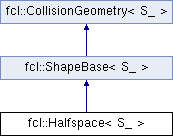FCL  0.6.0 Flexible Collision Library
fcl::Halfspace< S_ > Class Template Reference

Half Space: this is equivalent to the Planed in ODE. The separation plane is defined as n * x = d; Points in the negative side of the separation plane (i.e. {x | n * x < d}) are inside the half space and points in the positive side of the separation plane (i.e. {x | n * x > d}) are outside the half space. More...

`#include <halfspace.h>`

Inheritance diagram for fcl::Halfspace< S_ >:## Public Types

using S = S_Public Types inherited from fcl::ShapeBase< S_ >
using S = S_

## Public Member Functions

Halfspace (const Vector3< S > &n, S d)
Construct a half space with normal direction and offset.

Halfspace (S a, S b, S c, S d_)
Construct a plane with normal direction and offset.

signedDistance (const Vector3< S > &p) const

distance (const Vector3< S > &p) const

void computeLocalAABB () override
Compute AABB.

NODE_TYPE getNodeType () const override
Get node type: a half space.Public Member Functions inherited from fcl::ShapeBase< S_ >
OBJECT_TYPE getObjectType () const
Get object type: a geometric shape.Public Member Functions inherited from fcl::CollisionGeometry< S_ >
void * getUserData () const
get user data in geometry

void setUserData (void *data)
set user data in geometry

bool isOccupied () const
whether the object is completely occupied

bool isFree () const
whether the object is completely free

bool isUncertain () const
whether the object has some uncertainty

virtual Vector3< S_ > computeCOM () const
compute center of mass

virtual Matrix3< S_ > computeMomentofInertia () const
compute the inertia matrix, related to the origin

virtual S_ computeVolume () const
compute the volume

virtual Matrix3< S_ > computeMomentofInertiaRelatedToCOM () const
compute the inertia matrix, related to the com

## Public Attributes

Vector3< S > n
Planed normal.

d
Planed offset.Public Attributes inherited from fcl::CollisionGeometry< S_ >
Vector3< S_ > aabb_center
AABB center in local coordinate.

AABB< S_ > aabb_local
AABB in local coordinate, used for tight AABB when only translation transform.

void * user_data
pointer to user defined data specific to this object

S_ cost_density
collision cost for unit volume

S_ threshold_occupied
threshold for occupied ( >= is occupied)

S_ threshold_free

## Protected Member Functions

void unitNormalTest ()
Turn non-unit normal into unit.

## Detailed Description

### template<typename S_> class fcl::Halfspace< S_ >

Half Space: this is equivalent to the Planed in ODE. The separation plane is defined as n * x = d; Points in the negative side of the separation plane (i.e. {x | n * x < d}) are inside the half space and points in the positive side of the separation plane (i.e. {x | n * x > d}) are outside the half space.

The documentation for this class was generated from the following files:
• /home/js/dev/fcl/master/include/fcl/geometry/shape/halfspace.h
• /home/js/dev/fcl/master/include/fcl/geometry/shape/halfspace-inl.h